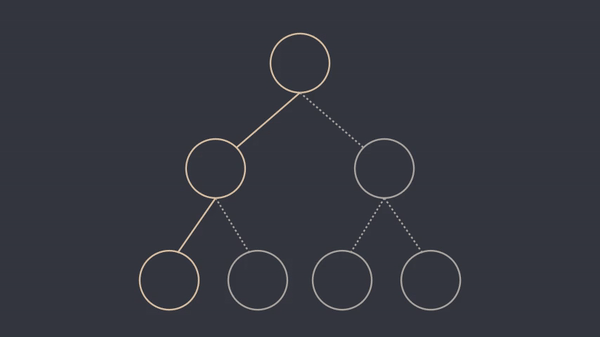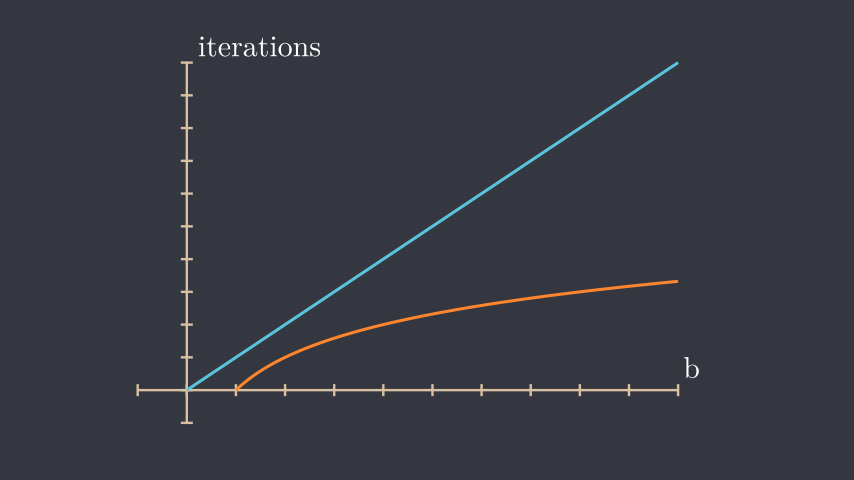# Fast Modular Exponentiation

20 August 2021

Motivation

Every now and then a problem arises that requires exponentiation, and usually, a naive approach to compute these values suffices. But every rule has its exceptions, so it can be helpful to know some tricks to ramp up efficiency. This is where fast modular exponentiation comes in, replacing the naive method and providing a much more efficient approach to the problem. The efficiency of such algorithms is crucial in areas such as cryptography and primality testing.

The naive approach

Let’s start by analysing the naive way of calculating $$a^b$$. The easiest and most intuitive way of doing this is simply multiplying the number $$a$$ by itself $$b-1$$ times.This method is very efficient for small values of $$a$$ and $$b$$ but if we need compute many large exponentiations it starts to become costly and not viable.

The fast approach

One might ask how it is even possible to optimize such a simple and central problem, and the answer lies in the arithmetical properties of exponentiation.

$$a^b = a^\frac{b}{2} \times a^\frac{b}{2}$$ $$a^\frac{b}{2} = a^\frac{b}{4} \times a^\frac{b}{4}$$

As we can see, it is possible to get $$a^\frac{b}{2}$$ by multiplying $$a^\frac{b}{4}$$ by itself and then multiplying $$a^\frac{b}{2}$$ by itself to get $$a^b$$. Notice how we got $$a^b$$ out of $$a^\frac{b}{4}$$ with two operations. Similiarly, we could compute $$a^b$$ using $$a^\frac{b}{8}$$ with three operations or $$a^\frac{b}{16}$$ with four operations. This shows that the number of operations required to compute $$a^b$$ remains linear as $$b$$ grows exponentially larger. In other words, the number of operations to compute $$a^b$$ increases logarithmically with $$b$$.As the animation suggests, we have turned this into a divide and conquer problem, where the base case (the leaves of the tree) is $$a$$. We can square the result at each step and reach the root node, which is $$a^b$$. The following animation shows this procedure for $$b=4$$.What if $$b$$ is not a power of two ?

You may have noticed that when we square the result at each step, we always get a power of two as the exponent, which is not ideal. Fortunately, there is a trick to adapt this algorithm to any positive integer.

$$a^{19} = a^{10011_2}$$ $$\: \: \: \: \: \: \: \: \: = a^{16 + 2 + 1}$$ $$\: \: \: \: \: \: \: \: \: \: \: \: \: \: \: \: \: \: \: \: \: = a^{16} \times a^{2} \times a^{1}$$

Since any integer can be represented as a sum of distinct powers of two, we can use the binary expansion of b to decide when to multiply the answer by the current result. If we iterate through the bits of the binary expansion of $$b$$ and the ith bit is 1, we proceed to multiply. The following animation shows this process to calculate $$2^{11}$$.Why modular ?

We have now seen how to compute exponentiation fast. But the name of the algorithm is fast modular exponentiation, not fast exponentiation, so where does the modular come from?
This is the last step of the algorithm and it is just as important to the rest if we want to preserve efficiency. Since in most cases we are checking what the remainder of dividing $$a^b$$ by a number $$c$$ is, there is no need to store the whole number. We can use the following modular property of numbers to keep the values smaller and preserve the answer.

$$(a \times b ) \: mod \: c = (a\: mod\: c \times b\: mod\: c)\: mod\: c$$
And therefore we can make an analogy to our problem and get the following expressions :

$$a^2 \: mod \: c = (a\: mod\: c \times a\: mod\: c)\: mod\: c$$ $$a^4 \: mod \: c = (a^2\: mod\: c \times a^2\: mod\: c)\: mod\: c$$
This is important because the larger the result becomes, the slower the iterations become due to the number of digits the numbers have. Both humans and computers have difficulty with large multiplications because the algorithms depend on the number of digits in the numbers.

The implementation

(Here is a C++ implementation of the algorithm)

int modPow(int base, int exp, int mod) {
while (exp != 0) {
if (exp & 1) { // if i-th bit is one multiply
}
base = (base * base) % mod; // increase at each iteration
exp = exp >> 1; //bitwise right shift
}

As we have already seen, this algorithm runs much faster than the naive approach. Since the problem is divided into two parts at each step, as shown in one of the previous animations, the number of operations is the height of the tree, and since we divide $$b$$ into two parts at each iteration, the answer becomes the number of times we have to divide a number $$b$$ until it becomes $$1$$ and this is $$\log_2 b$$ , hence we get a time complexity of $$O(log_2 b)$$, while the naive approach takes linear time $$O(b)$$.As $$b$$ gets larger the difference increases and that's why this algorithm is great for large exponentiations. For instance, if $$b=1\:000\:000$$ the naive approach takes $$\approx 1\:000\:000$$ operations while the fast approach takes $$\approx 20$$.